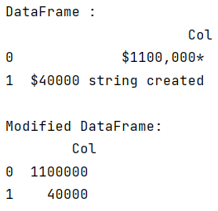# Python - Pandas applying regex to replace values

Given a Pandas DataFrame, we have to apply regex to replace values.
Submitted by Pranit Sharma, on July 30, 2022

Pandas is a special tool that allows us to perform complex manipulations of data effectively and efficiently. Inside pandas, we mostly deal with a dataset in the form of DataFrame. DataFrames are 2-dimensional data structures in pandas. DataFrames consist of rows, columns, and data.

Regex (Regular Expression)

A special format string is used for searching and filtering in pandas DataFrame rows.

The regex or a regular expression is simply a group of characters or special characters which follows a particular pattern with the help of which we can search and filter pandas DataFrame rows.

Example:

• 'K.*' : It will filter all the records which start with the letter 'K'.
• 'A.*' : It will filter all the records which start with the letter 'A'.

To replace a value using regex or by comparing the value by regex, we will use DataFrame[col].str.replace() method, inside which we will define our regex to compare.

Let us understand with the help of an example,

## Python code for applying regex to replace values

```# Importing pandas package
import pandas as pd

# Creating a dictionary
d = {'Col':['\$1100,000*','\$40000 string created']}

# Creating a dataframe
df = pd.DataFrame(d)

# Display Dataframe
print("DataFrame :\n",df,"\n")

# Using regex comparison
df['Col'] = df['Col'].str.replace(r'\D+', '', regex=True).astype('int')

# Display modified DataFrame
print("Modified DataFrame:\n",df)
```

Output:Languages: » C » C++ » C++ STL » Java » Data Structure » C#.Net » Android » Kotlin » SQL
Web Technologies: » PHP » Python » JavaScript » CSS » Ajax » Node.js » Web programming/HTML
Solved programs: » C » C++ » DS » Java » C#
Aptitude que. & ans.: » C » C++ » Java » DBMS
Interview que. & ans.: » C » Embedded C » Java » SEO » HR
CS Subjects: » CS Basics » O.S. » Networks » DBMS » Embedded Systems » Cloud Computing
» Machine learning » CS Organizations » Linux » DOS
More: » Articles » Puzzles » News/Updates